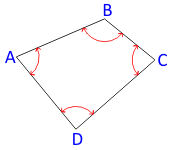## 1. Angle Condition

### a.Interior Angles

For a closed non-crossing loop traverse, the angle condition is:Equation B-1 Figure B-1 Angle Condtion - Loop A loop traverse which crosses itself doesn’t have conventional “interior” angles Eqn (B-1) won’t work. Some other angle condition must be established for such cases.Figure B-2 Crossling Traverse

### b. Deflection Angles

If deflection angles are used, the angle condition depends on how many times, if any, the traverse crosses itself.(a) Even crossings (b) Odd crossings Figure B-3 Deflection AnglesEquation B-2 Equation B-3

Angle condition equations are exact so the 0°, 180°, and 360° in the equations are also exact.

### c. Link Traverse

In order to determine angular misclosure for a closed link traverses, directions must be known at both ends:Figure B-4 Link Traverse Angle Condition

### Comments (0)

There are no comments posted here yet